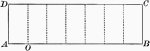### Rectangle Divided Into 7 sections

Rectangle divided into 7 sections.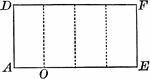### Rectangle Divided Into 4 sections

Rectangle divided into 4 sections.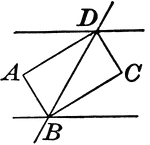### Rectangle Formed When Two Parallel Lines are Cut by a Transversal

Illustrations to show that if two parallel lines are cut by a transversal, the bisectors of the interior…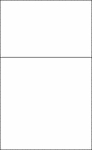### Golden Rectangle

Illustration showing the golden rectangle. Two quantities are considered to be in the golden ratio if…### Rectangle with Lengths a, b, and c with Areas ac and bc

An illustration of composite rectangle with lengths a, b, and c with area ac + bc. This illustration…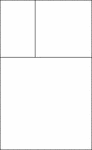### Golden Rectangles

Illustration showing a nesting of 2 golden rectangles. Two quantities are considered to be in the golden…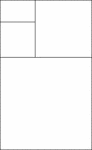### Golden Rectangles

Illustration showing a nesting of 3 golden rectangles. Two quantities are considered to be in the golden…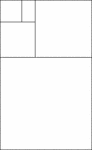### Golden Rectangles

Illustration showing a nesting of 4 golden rectangles. Two quantities are considered to be in the golden…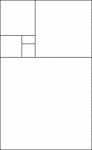### Golden Rectangles

Illustration showing a nesting of 5 golden rectangles. Two quantities are considered to be in the golden…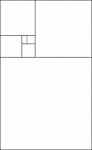### Golden Rectangles

Illustration showing a nesting of 6 golden rectangles. Two quantities are considered to be in the golden…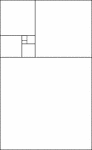### Golden Rectangles

Illustration showing a nesting of 7 golden rectangles. Two quantities are considered to be in the golden…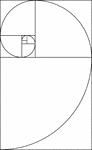### Golden Rectangles

Illustration showing succession of golden rectangles that are used to construct the golden spiral. Two…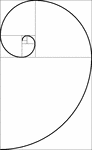### Golden Rectangles

Illustration showing succession of golden rectangles that are used to construct the golden spiral. Two…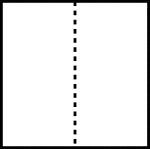### Divided Square

A square divided evenly by a dotted line.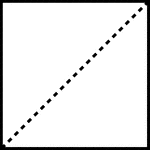### Divided Square

A square divided evenly by a dotted line, known as the diagonal.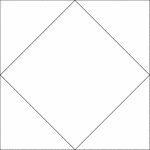### Square Inscribed In A Square

Illustration of a square inscribed in a square. The interior square is rotated 45° in relation to…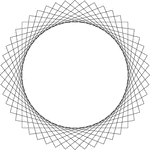### 12 Congruent Rotated Squares

Illustration of 12 congruent squares that have the same center. Each square has been rotated 7.5°…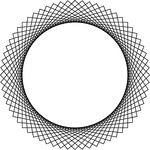### 16 Congruent Rotated Squares

Illustration of 16 congruent squares that have the same center. Each square has been rotated 5.625°…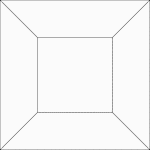### 2 Concentric Squares

Illustration of 2 concentric squares whose vertices are connected by line segments.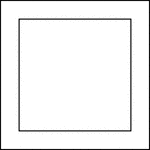### 2 Concentric Squares

Illustration of 2 concentric squares.### 2 Concentric Squares

Illustration of 2 concentric squares.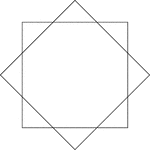### 2 Congruent Rotated Squares

Illustration of 2 congruent squares that have the same center. One square has been rotated 45° in…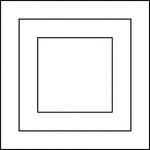### 3 Concentric Squares

Illustration of 3 concentric squares that are equally spaced.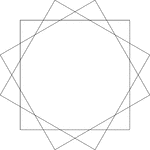### 3 Congruent Rotated Squares

Illustration of 3 congruent squares that have the same center. Each square has been rotated 30°…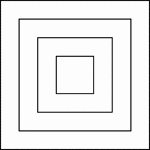### 4 Concentric Squares

Illustration of 4 concentric squares that are equally spaced.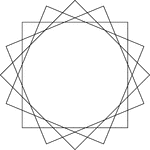### 4 Congruent Rotated Squares

Illustration of 4 congruent squares that have the same center. Each square has been rotated 22.5°…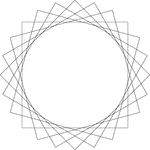### 6 Congruent Rotated Squares

Illustration of 6 congruent squares that have the same center. Each square has been rotated 15°…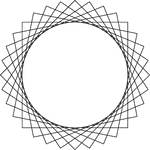### 8 Congruent Rotated Squares

Illustration of 8 congruent squares that have the same center. Each square has been rotated 11.25°…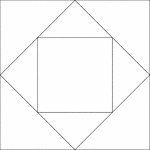### Squares Inscribed In Squares

Illustration of a square inscribed in a square that is inscribed in another square. Each successive…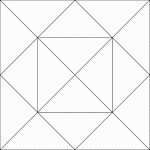### Squares Inscribed In Squares

Illustration of a square inscribed in a square that is inscribed in another square. Each successive…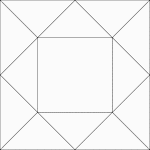### Squares Inscribed In Squares

Illustration of a square inscribed in a square that is inscribed in another square. Each successive…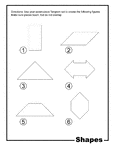### Shapes Outline Tangram Card

Outlines of shapes (rectangle, parallelogram, isosceles triangle, double arrow, rhombus, hexagon) made…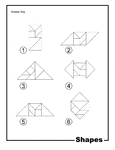### Shapes Outline Solution Tangram Card

Solutions for outlines of shapes (rectangle, parallelogram, isosceles triangle, double arrow, rhombus,…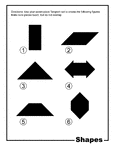### Shapes Silhouette Tangram Card

Silhouette outlines of shapes (rectangle, parallelogram, isosceles triangle, double arrow, rhombus,…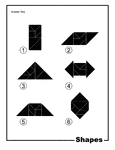### Shapes Silhouette Solution Tangram Card

Solutions for silhouette outlines of shapes (rectangle, parallelogram, isosceles triangle, double arrow,…